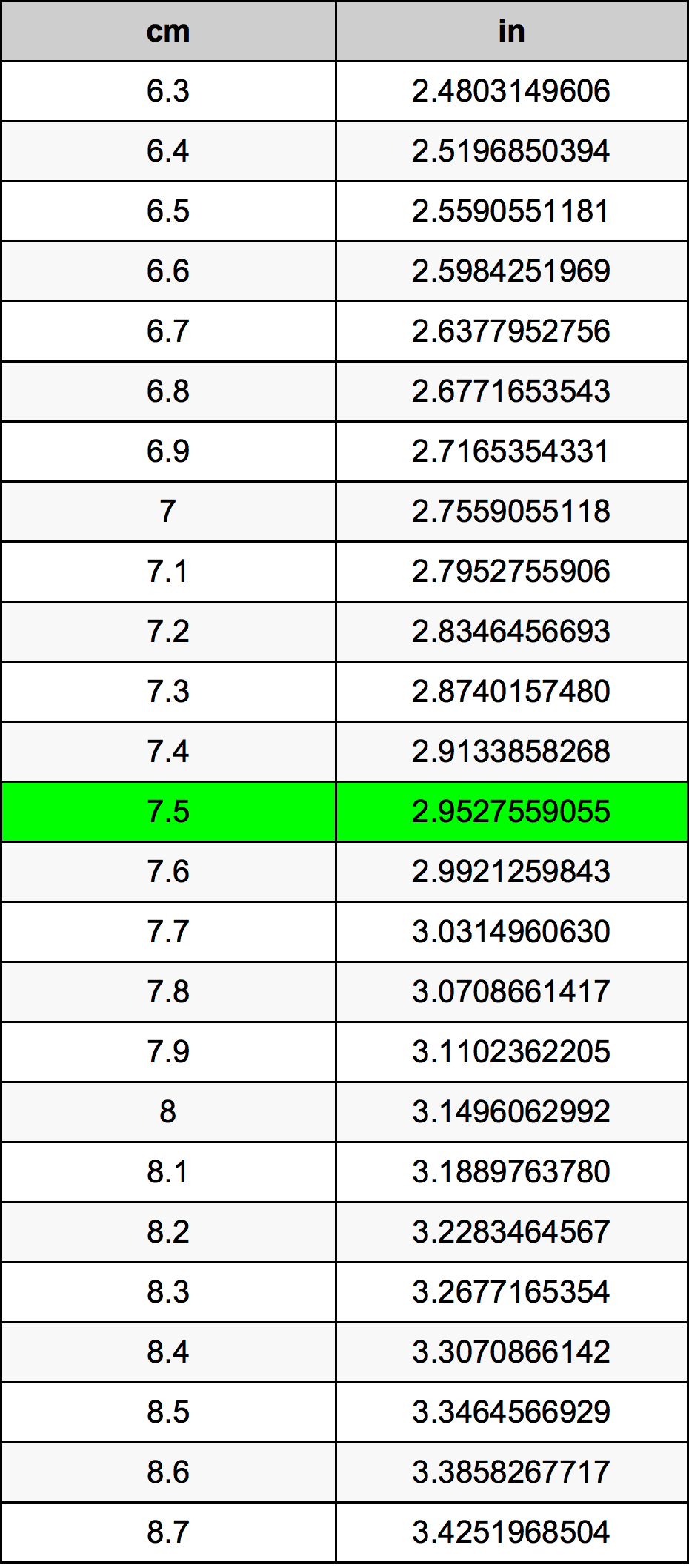Cm To Inches

# 7.5 cm to in7.5 Centimeters to Inches

cm
=
in

## How to convert 7.5 centimeters to inches?

 7.5 cm * 0.3937007874 in = 2.9527559055 in 1 cm
A common question is How many centimeter in 7.5 inch? And the answer is 19.05 cm in 7.5 in. Likewise the question how many inch in 7.5 centimeter has the answer of 2.9527559055 in in 7.5 cm.

## How much are 7.5 centimeters in inches?

7.5 centimeters equal 2.9527559055 inches (7.5cm = 2.9527559055in). Converting 7.5 cm to in is easy. Simply use our calculator above, or apply the formula to change the length 7.5 cm to in.

## Convert 7.5 cm to common lengths

UnitLength
Nanometer75000000.0 nm
Micrometer75000.0 µm
Millimeter75.0 mm
Centimeter7.5 cm
Inch2.9527559055 in
Foot0.2460629921 ft
Yard0.0820209974 yd
Meter0.075 m
Kilometer7.5e-05 km
Mile4.66028e-05 mi
Nautical mile4.04968e-05 nmi

## What is 7.5 centimeters in in?

To convert 7.5 cm to in multiply the length in centimeters by 0.3937007874. The 7.5 cm in in formula is [in] = 7.5 * 0.3937007874. Thus, for 7.5 centimeters in inch we get 2.9527559055 in.

## 7.5 Centimeter Conversion Table## Alternative spelling

7.5 Centimeter to in, 7.5 Centimeter in in, 7.5 Centimeter to Inch, 7.5 Centimeter in Inch, 7.5 cm to Inch, 7.5 cm in Inch, 7.5 cm to Inches, 7.5 cm in Inches, 7.5 Centimeter to Inches, 7.5 Centimeter in Inches, 7.5 cm to in, 7.5 cm in in, 7.5 Centimeters to Inch, 7.5 Centimeters in Inch